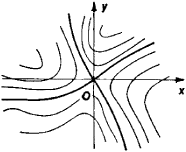(geology)
(mathematics)
A point where all the first partial derivatives of a function vanish but which is not a local maximum or minimum.
For a matrix of real numbers, an element that is both the smallest element of its row and the largest element of its column, or vice versa.
For a two-person, zero-sum game, an element of the payoff matrix that is the smallest element of its row and the largest element of its column, so that the corresponding strategies are optimal for each player, given the strategy chosen by the other player.

a critical point of a first-order differential equation. In a neighborhood of a saddle point, four half-line integralFigure 1

curves enter the critical point. Between the four curves there are four regions, each of which contains a family of integral curves resembling hyperbolas (see Figure l). The pattern of integral curves in a neighborhood of a saddle point is reminiscent of the contour lines of a hyperbolic paraboloid, which has the shape of a saddle—hence the name of the critical point.

References in periodicals archive ?
Bai, "Optimal parameters in the HSS-like methods for saddle-point problems," Numerical Linear Algebra with Applications, vol.
The solution of these problems is obtained by solving a large-scale linear system of saddle-point type.
By analysing the interactions between these opposit effects along the transition paths toward the equilibrium, our model shows that the economy converges to a stable saddle-point steady state with positive efficiency growth.
This result is in contrast to that of saddle-point equilibrium obtained in the previous studies, where the dynamic adjustment analysis of the long-term rate is around the LM curve after eliminating the short-term rate through the term structure.
The following saddle-point theorem is due to Silva ().
Also covered are theories from the work of Fisher, Craner, Le Cam, and others; the connection between saddle-point approximation and the Laplace method; and series convergence and the acceleration of that convergence.
If [delta] is greater than -[sigma]/(f - [lambda] - [sigma]), the dynamic system has a saddle-point equilibrium.
Moreover, the high steady state is saddle-point stable while the Laffer trap is locally indeterminate, so that sunspot equilibria occur near the low steady state.
Convexity and generalized convexity play a key role in many aspects of optimization, such as optimality conditions, saddle-point theorems, duality theorems, theorems of alternatives, and convergence of optimization algorithms, so the research on convexity and generalized convexity is one of the important aspects in mathematical programming.
Our results are motivated from uniqueness considerations of polynomial canonical representations of the phase or amplitude terms of oscillatory integrals encountered in the asymptotic analysis of the coefficients of mixed powers and multivariable generating functions via saddle-point methods.
We derive the saddle-point equations, and finally obtain at finite temperature
If this is the case then we can say that the model is saddle-point stable and it is possible to solve for the stable path.

Site: Follow: Share:
Open / Close